# Python Program to Find the Largest Among Three Numbers

In this tutorial you will learn about the Python Program to Find the Largest Among Three Numbers and its application with practical example.

In this tutorial, we will learn to create a Python Program to Find the Largest Among Three Numbers using Python programming.

## Prerequisites

Before starting with this tutorial we assume that you are best aware of the following Python programming topics:

• Basic Input and Output
• Basic Python  programming.
• Python if-else statements.
• Python Loop.

## Largest Among three?

In our program we will take three numbers  from user and stored in  variables a,b and c respectively. here we will use ‘if-elif-else’ ladder statement to find the largest among the three and display it.

## Python Program to Find the Largest Among Three Numbers

In this program we will Find Largest among three elements. We would first declared and initialized the required variables. then  we would prompt user to input values .Later we will find largest among three.

## Output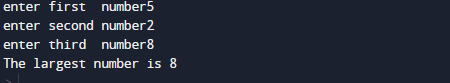In the above program, we have first declared and initialized a set variables required in the program.

• a = it will hold entered  first variable value.
• b = it will hold entered  second variable value.
• c = it will hold entered third variable value.

In our program, we will use 3 values in variable ‘a,b and c‘ ,after getting these values we will find the largest among  three.
First of all we declare variables and initialize value from user.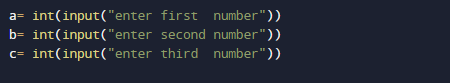After initiate values we will check condition for first variable has greatest value among three if the value in first variable is greater than second and third variable we can say that the value in first is largest among two.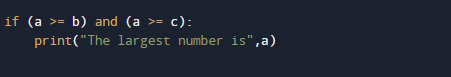if not we will move to elif section where we check second variable is greatest among two if it then we print second is the largest among these three.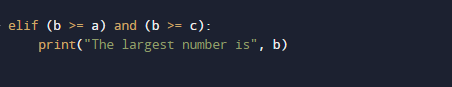else if we again jump to next ‘elif’ statement where we check renaming last variable is greatest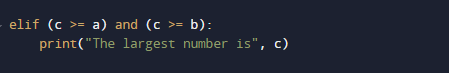if all these condition false it means the value within the variable is either zero or equal .Here use the ‘if…elif…else‘ ladder to find the largest among the three.

In this tutorial we have learn about the Python Program to Find the Largest Among Three Numbers and its application with practical example. I hope you will like this tutorial.